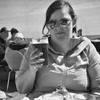# Correct answers are not counted.

I followed along with the video multiple times and keep running into the same problem. Even when I type the correct answers to the quiz, it always says I got "0 correct answers".

```var array = [
['What is the color of the sky?', 'blue'],
['What day is it?', 'friday']
];

var i;
var question;
var response;
var html;

function print(message) {
document.write(message);
}

for (i = 0; i < array.length; i += 1) {
question = array[i];
response = prompt(question);
response = response.toLowerCase;
}
}

html = "You got " + correctAnswers + " question(s) right.";
print(html);
```